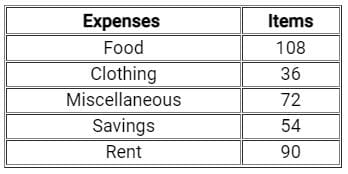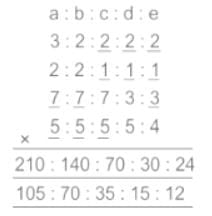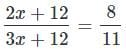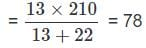SAT  >  Test: Ratio & Proportion

# Test: Ratio & Proportion

Test Description

## 15 Questions MCQ Test Mathematics for SAT | Test: Ratio & Proportion

Test: Ratio & Proportion for SAT 2023 is part of Mathematics for SAT preparation. The Test: Ratio & Proportion questions and answers have been prepared according to the SAT exam syllabus.The Test: Ratio & Proportion MCQs are made for SAT 2023 Exam. Find important definitions, questions, notes, meanings, examples, exercises, MCQs and online tests for Test: Ratio & Proportion below.
Solutions of Test: Ratio & Proportion questions in English are available as part of our Mathematics for SAT for SAT & Test: Ratio & Proportion solutions in Hindi for Mathematics for SAT course. Download more important topics, notes, lectures and mock test series for SAT Exam by signing up for free. Attempt Test: Ratio & Proportion | 15 questions in 25 minutes | Mock test for SAT preparation | Free important questions MCQ to study Mathematics for SAT for SAT Exam | Download free PDF with solutions
 1 Crore+ students have signed up on EduRev. Have you?
Test: Ratio & Proportion - Question 1

### The sum of 3 numbers is 285. Ratio between 2nd and 3rd numbers is 6 ∶ 5. Ratio between 1st and 2nd numbers is 3 ∶ 7. The 3rd number is?

Detailed Solution for Test: Ratio & Proportion - Question 1

Given:

The sum of 3 numbers is 285. Ratio between 2nd and 3rd numbers is 6 ∶ 5.

Ratio between 1st and 2nd numbers is 3 ∶ 7.

Calculation:

Let the 1st number be x

Let the 2nd number be y

Let the 3rd number be z

The sum of 3rd number is 285

According to the question

Ratio of 2nd and 3rd number

⇒ y : z = 6 : 5

Ratio of 1th and 2nd number

⇒ x : y = 3 : 7

Ratio of x : y : z

⇒ (x × y) : (y × y) : (y × z) = (3 × 6) : (7 × 6) : (7 × 5)

⇒ x : y : z = 18 : 42 : 35

The 3rd number z = 285 × 35 /(18 + 42 + 35)

⇒ z = 285 × 35 / 95 = 105

∴   The 3rd number is 105

Test: Ratio & Proportion - Question 2

### The ratio of average of expenses on food, clothing and miscellaneous items to the average of expenses on savings and rent isDetailed Solution for Test: Ratio & Proportion - Question 2

(108 + 36 + 72) ∶ (54 + 90)

= 216 ∶ 144

= 3 ∶ 2

Test: Ratio & Proportion - Question 3

### If a2 + b2 + c2 - ab - bc - ca = 0, then a ∶ b ∶ c is:

Detailed Solution for Test: Ratio & Proportion - Question 3

Given :

Equation is a2 + b2 + c2 - ab - bc - ca = 0

Solution :

Here we have 3 variables and only one equation .

Note  To solve these type of question we assume the value of any 2 variables.

Let assume that b = 1 and C = 1

⇒ a+ 1 + 1 - a - 1 - a = 0

⇒ a2 + 1 - 2a = 0

⇒ ( a - 1 )2 = 0  [ ∵  ( A+B )2 = A2 + B2 + 2AB ]

Now a = 1

Now we have a = 1 , b = 1 and c = 1

So a : b : c = 1 : 1 : 1

Hence the correct answer is "1 : 1 : 1".

Test: Ratio & Proportion - Question 4

A man has 25 paise, 50 paise and 1 Rupee coins. There are 220 coins in all and the total amount is 160. If there are thrice as many 1 Rupee coins as there are 25 paise coins, then what is the number of 50 paise coins?

Detailed Solution for Test: Ratio & Proportion - Question 4

Given:

Total coin = 220

Total money = Rs. 160

There are thrice as many 1 Rupee coins as there are 25 paise coins.

Concept used:

Ratio method is used.

Calculation:

Let the 25 paise coins be 'x'

So, one rupees coins = 3x

50 paise coins = 220 – x – (3x) = 220 – (4x)

According to the questions,

3x + [(220 – 4x)/2] + x/4 =160

⇒ (12x + 440 – 8x + x)/4 = 160

⇒  5x + 440 = 640

⇒ 5x = 200

⇒ x = 40

So, 50 paise coins = 220 – (4x) = 220 – (4 × 40) = 60

∴ The number of 50 paise coin is 60.

Test: Ratio & Proportion - Question 5

If a : b = 3 : 2, b : c = 2 : 1, c : d = 1/3 : 1/7 and d : e = 1/4 : 1/5 find a : b : c : d : e.

Detailed Solution for Test: Ratio & Proportion - Question 5

Given :

(i) a : b = 3 : 2,

(ii) b : c= 2 : 1,

(iii) c : d = 1/3 : 1/7,

(iv) d : e = 1/4 : 1/5

Calculations:

To solve these type of questions fill the blank by side values and then multiply all the ratiosShortcut Trick:
a : b = 3 : 2 now check which options satisfied this ratio

1) a : b = 100 : 75 = 4 : 3 not equal to 3 : 2

2) a : b = 100 : 30 = 10 : 3 not equal to 3 : 2

3) a : b = 105 : 70 = 21 : 14 = 3 : 2 which is equal to 3 : 2

4) a : b = 105 : 35 = 21 : 7 = 3 : 1 which is not equal to 3 : 2

Hence option c is correct option

Test: Ratio & Proportion - Question 6

u : v = 4 : 7 and v : w = 9 : 7. If u = 72, then what is the value of w?

Detailed Solution for Test: Ratio & Proportion - Question 6

Given:

u : v = 4 : 7 and v : w = 9 : 7

Concept Used: In this type of question, number can be calculated by using the below formulae

Formula Used: if u ∶ v = a ∶ b, then u × b = v × a.

Calculation:

u : v = 4 : 7 and v : w = 9 : 7

To make ratio v equal in both cases

We have to multiply the 1st ratio by 9 and 2nd ratio by 7

u : v = 9 × 4 : 9 × 7 = 36 : 63    ----(i)

v : w = 9 × 7 : 7 × 7 = 63 : 49    ----(ii)

Form (i) and (ii), we can see that the ratio v is equal in both cases

So, Equating the ratios we get,

u ∶ v ∶ w = 36 ∶ 63 ∶ 49

⇒ u ∶ w = 36 ∶ 49

When u = 72,

⇒ w = 49 × 72/36 = 98

∴ Value of w is 98

Test: Ratio & Proportion - Question 7

A diamond weighing 35 grams cost Rs. 12,250 is cut down into two pieces having weights in the ratio of 5 ∶ 2. If the price directly proportional to the square of the weight then find the loss incurred.

Detailed Solution for Test: Ratio & Proportion - Question 7

Given:

New ratio is 5 ∶ 2.

The price of diamond before broken is Rs. 12250.

Concept used:

Using the concept simple ratio.

Calculation:

Let the weight of each piece of diamond be 5x and 2x.

Total weight of diamond = 5x + 2x = 7x

Cost of the diamond = (7x)2 = 49x2

Cost of first piece = (5x)2 = 25x2

Cost of second piece = (2x)2 = 4x2

Total cost of diamond after weight = 25x2 + 4x2 = 29x2

⇒ New cost = 29x2

According to the question,

49x2 = 12250

⇒ x2 = 250

The loss in the price of diamond after broken = 49x2 – 29x2

⇒ The required loss = 20x2

⇒ 250 × 20

⇒ Rs. 5000

∴ The loss in the price of diamond after broken is Rs. 5000.

Test: Ratio & Proportion - Question 8

In a bag, there are coins of 5ps, 10ps, and 25ps in a ratio of 3 : 2 : 1. If there are Rs. 60 in all, how many 5ps coins are there?

Detailed Solution for Test: Ratio & Proportion - Question 8

Given:

5p : 10p : 25p = 3 : 2 : 1 = 3x : 2x : x

Concept:

1 Rupee = 100 paise

Calculation:

60 Rupees = 60 × 100 = 6000 paise

⇒ 5 × 3x + 10 × 2x + 25 × 1x = 6000

⇒ 15x + 20x + 25x = 6000

⇒ 60x = 6000

⇒ x = 100

∴ Number of 5 paise coins = 3x = 3 × 100 = 300

Test: Ratio & Proportion - Question 9

Rs.750 are divided among A, B and C in such a manner that A : B is 5 : 2 and B : C is 7 : 13. What is A’s share?

Detailed Solution for Test: Ratio & Proportion - Question 9

Given:

Total rupees = Rs 750

Calculation:

A : B = 5 : 2

B : C = 7 : 13

A : B : C = 5 × 7 : 2 × 7 : 2 × 13 = 35 : 14 : 26

Total Sum = 750

⇒ 35 x + 14x + 26x = 750

⇒ x = 10

So, A's share = 35 × 10 = Rs 350

∴ The required answer is Rs 350

Test: Ratio & Proportion - Question 10

In a family, the age of father, mother, son, and grandson are A, B, C, and D respectively. Given that A - B = 3, B + C = 78, C + D = 33 and the average age of the family is 34 years, then (B - C) is:

Detailed Solution for Test: Ratio & Proportion - Question 10

Given:

A - B = 3, B + C = 78, C + D = 33 and the average age of the family is 34 years

Formula used:

Total age = Average age × total number of peoples

Calculation:​

Total age of the family = 4 × 34 = 136 years

A + B + C + D = 136

⇒ A + B = 136 – 33     (∵ C + D = 33)

A + B = 103 years      ---- (i)

A – B = 3 years (Given)      ----- (ii)

From (i) and (ii)

2A = 106 years

∴ A = 53 years

B = 103 – 53 = 50 years    ( from i )

Also C + B = 78    (Given)

⇒ C = 78 – 50 = 28 years

∴ B – C = 50 – 28 = 22 years

Test: Ratio & Proportion - Question 11

The monthly salaries of A and B are in the ratio 5 : 6. If both of them get a salary increment of Rs. 2000, the new ratio becomes 11 : 13. What is the new monthly salary of A?

Detailed Solution for Test: Ratio & Proportion - Question 11

Given:

Ratio of salaries of A and B = 5 : 6

New ratio = 11 : 13

Salary increment of each = Rs. 2000

Calculations:

Let the unit of ratio be x

A’s salary = 5x

B’s salary = 6x

After increment,

⇒ (5x + 2000)/(6x + 2000) = 11/13

⇒ 13(5x + 2000) = 11(6x + 2000)

⇒ 65x + 26000 = 66x + 22000

⇒ x = Rs. 4000

⇒ A’s new month salary = 5x + 2000 = 5 × 4000 + 2000

⇒ A’s new month salary = Rs. 22000

∴ The new monthly salary of A is Rs. 22000.

Test: Ratio & Proportion - Question 12

Rahul has a bag which contains Rs. 1, 50 paisa, and 25 paisa coins and the ratio of number of coins is 1 ∶ 1/2 ∶ 1/3. If Rahul has a total amount of Rs 1120, then find the total value of 25 paisa coins.

Detailed Solution for Test: Ratio & Proportion - Question 12

Given:

Total amount = Rs 1120

Ratio of coins of Rs 1, 50 paisa, and 25 paisa = 1 ∶ 1/2 ∶ 1/3

Formula Used:

Value of coins = Number of coins × per coin value

Calculation:

We know that

Rs 1 = 100 paisa

Ratio of value per coin = 100 ∶ 50 ∶ 25

⇒ 4 ∶ 2 ∶ 1

Ratio of number of coins = 1 ∶ 1/2 ∶ 1/3

⇒ 6 ∶ 3 ∶ 2

Total value of coins = 24 ∶ 6 ∶ 2

⇒ 12 ∶ 3 ∶ 1 = 16 units

⇒ 16 units = 1120

⇒ 1 units = 70

Value of 25 paisa coins = 1 units

⇒ 1 × 70

⇒ Rs. 70

The total number of 25 paisa coins is Rs. 70.

Test: Ratio & Proportion - Question 13

A man divided an amount between his sons in the ratio of their ages. The sons received Rs. 54000 and Rs. 48000. If one son is 5 years older than the other, find the age of the younger son.

Detailed Solution for Test: Ratio & Proportion - Question 13

Let the son’s ages be ‘x’ years and ‘y’ years

Given, x - y = 5

⇒ x = y + 5

Now,

Ratio of their shares = ratio of their ages

⇒ 54000 ∶ 48000 = x ∶ y

⇒ 9 ∶ 8 = (y + 5) ∶ y

⇒ 9y = 8y + 40

⇒ y = 40

∴ The younger son’s age is 40 years

Test: Ratio & Proportion - Question 14

The number of students in 3 classes is in the ratio 2 : 3 : 4. If 12 students are increased in each class this ratio changes to 8 : 11 : 14. The total number of students in the three classes in the beginning was

Detailed Solution for Test: Ratio & Proportion - Question 14

Let the number of students in the classes be 2x, 3x and 4x respectively;
Total students = 2x + 3x + 4x = 9x
According to the question,or, 24x + 96 = 22x + 132
or, 2x = 132 − 96 or,
x = 36/2 = 18
Hence,Original number of students,
9x = 9 × 18
= 162

Test: Ratio & Proportion - Question 15

A box has 210 coins of denominations one-rupee and fifty paise only. The ratio of their respective values is 13 : 11. The number of one-rupee coin is

Detailed Solution for Test: Ratio & Proportion - Question 15

Respective ratio of the Number of coins;
= 13 : 11 × 2 = 13 : 22
Hence, Number of 1 rupee coins;## Mathematics for SAT

172 videos|94 docs|66 tests
 Use Code STAYHOME200 and get INR 200 additional OFF Use Coupon Code
Information about Test: Ratio & Proportion Page
In this test you can find the Exam questions for Test: Ratio & Proportion solved & explained in the simplest way possible. Besides giving Questions and answers for Test: Ratio & Proportion, EduRev gives you an ample number of Online tests for practice

## Mathematics for SAT

172 videos|94 docs|66 tests